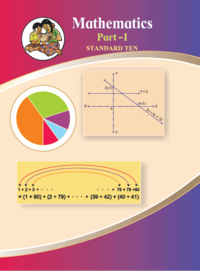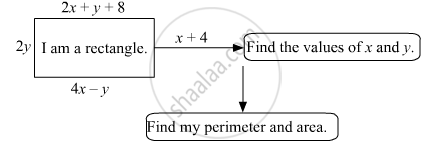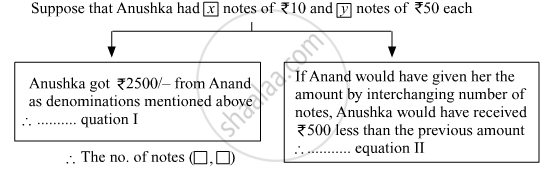# Balbharati solutions for Mathematics 1 Algebra 10th Standard SSC Maharashtra State Board chapter 1 - Linear Equations in Two Variables [Latest edition]

#### Chapters## Chapter 1: Linear Equations in Two Variables

Practice Set 1.1Practice Set 1.2Practice Set 1.3Practice Set 1.4Practice Set 1.5Problem Set 1
Practice Set 1.1 [Pages 4 - 5]

### Balbharati solutions for Mathematics 1 Algebra 10th Standard SSC Maharashtra State Board Chapter 1 Linear Equations in Two Variables Practice Set 1.1 [Pages 4 - 5]

Practice Set 1.1 | Q 1 | Page 4

Complete the following activity to solve the simultaneous equations.
5x + 3y = 9 -----(I)
2x + 3y = 12 ----- (II)

Practice Set 1.1 | Q 2.1 | Page 5

Solve the following simultaneous equation.
3a + 5b = 26; a + 5b = 22

Practice Set 1.1 | Q 2.2 | Page 5

Solve the following simultaneous equation.
x + 7y = 10; 3x – 2y = 7

Practice Set 1.1 | Q 2.3 | Page 5

Solve the following simultaneous equation.
2x – 3y = 9; 2x + y = 13

Practice Set 1.1 | Q 2.4 | Page 5

Solve the following simultaneous equation.
5m – 3n = 19; m – 6n = –7

Practice Set 1.1 | Q 2.5 | Page 5

Solve the following simultaneous equation.
5x + 2y = –3; x + 5y = 4

Practice Set 1.1 | Q 2.6 | Page 5

Solve the following simultaneous equation.
$\frac{1}{3}x + y = \frac{10}{3}; 2x + \frac{1}{4}y = \frac{11}{4}$

Practice Set 1.1 | Q 2.7 | Page 5

Solve the following simultaneous equation.
99x + 101y = 499; 101x + 99y = 501

Practice Set 1.1 | Q 2.8 | Page 5

Solve the following simultaneous equation.
49x – 57y = 172; 57x – 49y = 252

Practice Set 1.2 [Page 8]

### Balbharati solutions for Mathematics 1 Algebra 10th Standard SSC Maharashtra State Board Chapter 1 Linear Equations in Two Variables Practice Set 1.2 [Page 8]

Practice Set 1.2 | Q 1 | Page 8

Complete the following table to draw graph of the equations - (I) x + y = 3 (II) x – y = 4

x + y = 3

 x 3 y 5 3 (x,y) (3,0) (0,3)

x – y = 4

 x -1 0 y 0 -4 (x,y) (0,-4)
Practice Set 1.2 | Q 2.1 | Page 8

Solve the following simultaneous equations graphically.
= 6 ; – = 4

Practice Set 1.2 | Q 2.2 | Page 8

Solve the following simultaneous equations graphically.
x + y = 5 ; x – y = 3

Practice Set 1.2 | Q 2.3 | Page 8

Solve the following simultaneous equations graphically.
x + y = 0 ; 2x – y = 9

Practice Set 1.2 | Q 2.4 | Page 8

Solve the following simultaneous equations graphically.
3x – y = 2 ; 2x – y = 3

Practice Set 1.2 | Q 2.5 | Page 8

Solve the Following Simultaneous Equations Graphically.

3x – 4y = –7 ; 5x – 2y = 0

Practice Set 1.2 | Q 2.6 | Page 8

Solve the following simultaneous equations graphically.

2x – 3y = 4 ; 3y – x = 4

Practice Set 1.3 [Page 16]

### Balbharati solutions for Mathematics 1 Algebra 10th Standard SSC Maharashtra State Board Chapter 1 Linear Equations in Two Variables Practice Set 1.3 [Page 16]

Practice Set 1.3 | Q 1 | Page 16

Fill in the blanks with correct number$\begin{vmatrix}3 & 2 \\ 4 & 5\end{vmatrix}$ = 3 x ____ - ____ x 4 = ____ - 8 = ____

Practice Set 1.3 | Q 2.1 | Page 16

Find the values of following determinant.

$\begin{vmatrix}- 1 & 7 \\ 2 & 4\end{vmatrix}$
Practice Set 1.3 | Q 2.2 | Page 16

Find the values of following determinant.

$\begin{vmatrix}5 & 3 \\ - 7 & 0\end{vmatrix}$
Practice Set 1.3 | Q 2.3 | Page 16

Find the values of following determinant.

$\begin{vmatrix}\frac{7}{3} & \frac{5}{3} \\ \frac{3}{2} & \frac{1}{2}\end{vmatrix}$
Practice Set 1.3 | Q 3.1 | Page 16

Solve the following simultaneous equations using Cramer’s rule.
3x – 4y = 10 ; 4x + 3y = 5

Practice Set 1.3 | Q 3.2 | Page 16

Solve the following simultaneous equations using Cramer’s rule.
4x + 3y – 4 = 0 ; 6x = 8 – 5y

Practice Set 1.3 | Q 3.3 | Page 16

Solve the following simultaneous equations using Cramer’s rule.
x + 2y = –1 ; 2x – 3y = 12

Practice Set 1.3 | Q 3.4 | Page 16

Solve the following simultaneous equations using Cramer’s rule.
6x – 4y = –12 ; 8x – 3y = –2

Practice Set 1.3 | Q 3.5 | Page 16

Solve the following simultaneous equations using Cramer’s rule.
4m + 6n = 54 ; 3m + 2n = 28

Practice Set 1.3 | Q 3.6 | Page 16

Solve the following simultaneous equations using Cramer’s rule.
2x + 3y = 2; x - y/2 = 1/2.

Practice Set 1.4 [Page 19]

### Balbharati solutions for Mathematics 1 Algebra 10th Standard SSC Maharashtra State Board Chapter 1 Linear Equations in Two Variables Practice Set 1.4 [Page 19]

Practice Set 1.4 | Q 1.1 | Page 19

Solve the following simultaneous equations.
$\frac{2}{x} - \frac{3}{y} = 15; \frac{8}{x} + \frac{5}{y} = 77$

Practice Set 1.4 | Q 1.2 | Page 19

Solve the following simultaneous equations.
$\frac{10}{x + y} + \frac{2}{x - y} = 4; \frac{15}{x + y} - \frac{5}{x - y} = - 2$

Practice Set 1.4 | Q 1.3 | Page 19

Solve the following simultaneous equations.

27/(x -2) + 31/(y + 3) = 85, 31/(x -2) + 27/(y + 3) = 89.

Practice Set 1.4 | Q 1.4 | Page 19

Solve the following simultaneous equation.

1/(3x + y) + 1/(3x - y) = 3/4; 1/(2(3x + y))  - 1/(2(3x - y))  = -1/8

Practice Set 1.5 [Page 26]

### Balbharati solutions for Mathematics 1 Algebra 10th Standard SSC Maharashtra State Board Chapter 1 Linear Equations in Two Variables Practice Set 1.5 [Page 26]

Practice Set 1.5 | Q 1 | Page 26

Two numbers differ by 3. The sum of twice the smaller number and thrice the greater number is 19. Find the numbers.

Practice Set 1.5 | Q 2 | Page 26

Complete the following.Practice Set 1.5 | Q 3 | Page 26

The sum of father’s age and twice the age of his son is 70. If we double the age of the father and add it to the age of his son the sum is 95. Find their present ages.

Practice Set 1.5 | Q 4 | Page 26

The denominator of a fraction is 4 more than twice its numerator. Denominator becomes 12 times the numerator, if both the numerator and the denominator are reduced by 6. Find the fraction.

Practice Set 1.5 | Q 5 | Page 26

Two types of boxes A, B are to be placed in a truck having a capacity of 10 tons. When 150 boxes of type A and 100 boxes of type B are loaded in the truck, it weighes 10 tons. But when 260 boxes of type A are loaded in the truck, it can still accommodate 40 boxes of type B, so that it is fully loaded. Find the weight of each type of box.

Practice Set 1.5 | Q 6 | Page 26

Out of 1900 km, Vishal travelled some distance by bus and some by aeroplane. The bus travels with an average speed of 60 km/hr and the average speed of the aeroplane is 700 km/hr. It takes 5 hours to complete the journey. Find the distance, Vishal travelled by bus.

Problem Set 1 [Pages 27 - 29]

### Balbharati solutions for Mathematics 1 Algebra 10th Standard SSC Maharashtra State Board Chapter 1 Linear Equations in Two Variables Problem Set 1 [Pages 27 - 29]

Problem Set 1 | Q 1.1 | Page 27

Choose correct alternative for the following question.
To draw graph of 4x + 5y = 19, Find y when x = 1.

• 4

• 3

• 2

• -3

Problem Set 1 | Q 1.2 | Page 27

Choose correct alternative for the following question.
For simultaneous equations in variables x and y, D= 49, Dy = –63, D = 7 then what is x ?

• 7

• -7

• 1/7

• (-1)/7

Problem Set 1 | Q 1.3 | Page 27

Choose correct alternative for the following question.
Find the value of $\begin{vmatrix}5 & 3 \\ - 7 & - 4\end{vmatrix}$

• –1

• –41

• 41

• 1

Problem Set 1 | Q 1.4 | Page 27

Choose correct alternative for the following question.
To solve x + y = 3 ; 3x – 2y – 4 = 0 by determinant method find D.

(A) 5
(B) 1
(C) -5
(D) -1

Problem Set 1 | Q 1.5 | Page 27

Choose correct alternative for the following question.
ax + by = c and mx + ny = d and an ≠ bm then these simultaneous equations have -

• Only one common solution.

• No solution.

• Infinite number of solutions.

• Only two solution.

Problem Set 1 | Q 2 | Page 27

Complete the following table to draw the graph of 2x –  6y = 3

 x -5 x y x 0 (x,y) (-5,x) (x,0)
Problem Set 1 | Q 3.1 | Page 27

Solve the following simultaneous equation graphically.
2x + 3y = 12 ; x – y = 1

Problem Set 1 | Q 3.2 | Page 27

Solve the following simultaneous equation graphically.
x – 3y = 1 ; 3x – 2y + 4 = 0

Problem Set 1 | Q 3.3 | Page 27

Solve the following simultaneous equation graphically.
5x – 6y + 30 = 0 ; 5x + 4y – 20 = 0

Problem Set 1 | Q 3.4 | Page 27

Solve the following simultaneous equation graphically.
3x – y – 2 = 0 ; 2x + y = 8

Problem Set 1 | Q 3.5 | Page 27

Solve the following simultaneous equation graphically.
3x + y = 10 ;  x – y = 2

Problem Set 1 | Q 4.1 | Page 27

Find the value of the following determinant.

$\begin{vmatrix}4 & 3 \\ 2 & 7\end{vmatrix}$
Problem Set 1 | Q 4.2 | Page 27

Find the value of the following determinant.

$\begin{vmatrix}5 & - 2 \\ - 3 & 1\end{vmatrix}$
Problem Set 1 | Q 4.3 | Page 27

Find the value of the following determinant.

$\begin{vmatrix}3 & - 1 \\ 1 & 4\end{vmatrix}$
Problem Set 1 | Q 5.1 | Page 28

Solve the following equations by Cramer’s method.
6x – 3y = –10 ; 3x + 5y – 8 = 0

Problem Set 1 | Q 5.2 | Page 28

Solve the following equation by Cramer’s method.
4m – 2n = –4 ; 4m + 3n = 16

Problem Set 1 | Q 5.3 | Page 28

Solve the following equations by Cramer’s method.
3x – 2= $\frac{5}{2}$ ; $\frac{1}{3}x + 3y = - \frac{4}{3}$

Problem Set 1 | Q 5.4 | Page 28

Solve the following equations by Cramer’s method.
7x + 3y = 15 ; 12y – 5x = 39

Problem Set 1 | Q 5.5 | Page 28

Solve the following equations by Cramer’s method.
$\frac{x + y - 8}{2} = \frac{x + 2y - 14}{3} = \frac{3x - y}{4}$

Problem Set 1 | Q 6.1 | Page 28

Solve the following simultaneous equations.
$\frac{2}{x} + \frac{2}{3y} = \frac{1}{6} ; \frac{3}{x} + \frac{2}{y} = 0$

Problem Set 1 | Q 6.2 | Page 28

Solve the following simultaneous equations.
$\frac{7}{2x + 1} + \frac{13}{y + 2} = 27 ; \frac{13}{2x + 1} + \frac{7}{y + 2} = 33$

Problem Set 1 | Q 6.3 | Page 28

Solve the following simultaneous equations.

$\frac{148}{x} + \frac{231}{y} = \frac{527}{xy} ; \frac{231}{x} + \frac{148}{y} = \frac{610}{xy}$
Problem Set 1 | Q 6.4 | Page 28

Solve the following simultaneous equations.

$\frac{7x - 2y}{xy} = 5 ; \frac{8x + 7y}{xy} = 15$
Problem Set 1 | Q 6.5 | Page 28

Solve the following simultaneous equations.

$\frac{1}{2\left( 3x + 4y \right)} + \frac{1}{5\left( 2x - 3y \right)} = \frac{1}{4} ; \frac{5}{\left( 3x + 4y \right)} - \frac{2}{\left( 2x - 3y \right)} = - \frac{3}{2}$
Problem Set 1 | Q 7.1 | Page 28

Solve the following word problem.
A two digit number and the number with digits interchanged add up to 143. In the given number the digit in unit’s place is 3 more than the digit in the ten’s place. Find the original number.

Problem Set 1 | Q 7.2 | Page 29

Solve the following word problem.
Kantabai bought $1\frac{1}{2}$ kg tea and 5 kg sugar from a shop. She paid Rs 50 as return fare for rickshaw. Total expense was Rs 700. Then she realised that by ordering online the goods can be bought with free home delivery at the same price. So next month she placed the order online for 2 kg tea and 7 kg sugar. She paid Rs 880 for that. Find the rate of sugar and tea per kg.

Problem Set 1 | Q 7.3 | Page 29

Solve the following word problem.
To find number of notes that Anushka had, complete the following activity.Problem Set 1 | Q 7.4 | Page 29

Solve the Following Word Problem.
Sum of the present ages of Manish and Savita is 31. Manish’s age 3 years ago was 4 times the age of Savita. Find their present ages.

Problem Set 1 | Q 7.5 | Page 29

Solve the Following Word Problem.
In a factory the ratio of salary of skilled and unskilled workers is 5 : 3. Total salary of one day of both of them is Rs 720. Find daily wages of skilled and unskilled workers.

Problem Set 1 | Q 7.6 | Page 29

Solve the Following Word Problem.
Places A and B are 30 km apart and they are on a st raight road. Hamid travels from A to B on bike. At the same time Joseph starts from B on bike, travels towards A. They meet each other after 20 minutes. If Joseph would have started from B at the same time but in the opposite direction (instead of towards A) Hamid would have caught him after 3 hours. Find the speed of Hamid and Joseph.

## Chapter 1: Linear Equations in Two Variables

Practice Set 1.1Practice Set 1.2Practice Set 1.3Practice Set 1.4Practice Set 1.5Problem Set 1## Balbharati solutions for Mathematics 1 Algebra 10th Standard SSC Maharashtra State Board chapter 1 - Linear Equations in Two Variables

Balbharati solutions for Mathematics 1 Algebra 10th Standard SSC Maharashtra State Board chapter 1 (Linear Equations in Two Variables) include all questions with solution and detail explanation. This will clear students doubts about any question and improve application skills while preparing for board exams. The detailed, step-by-step solutions will help you understand the concepts better and clear your confusions, if any. Shaalaa.com has the Maharashtra State Board Mathematics 1 Algebra 10th Standard SSC Maharashtra State Board solutions in a manner that help students grasp basic concepts better and faster.

Further, we at Shaalaa.com provide such solutions so that students can prepare for written exams. Balbharati textbook solutions can be a core help for self-study and acts as a perfect self-help guidance for students.

Concepts covered in Mathematics 1 Algebra 10th Standard SSC Maharashtra State Board chapter 1 Linear Equations in Two Variables are Linear Equations in Two Variables Applications, Cramer'S Rule, Cross - Multiplication Method, Substitution Method, Elimination Method, Equations Reducible to a Pair of Linear Equations in Two Variables, Simple Situational Problems, Inconsistency of Pair of Linear Equations, Consistency of Pair of Linear Equations, Graphical Method of Solution of a Pair of Linear Equations, Determinant of Order Two, Pair of Linear Equations in Two Variables, Linear Equations in Two Variables.

Using Balbharati 10th Standard Board Exam solutions Linear Equations in Two Variables exercise by students are an easy way to prepare for the exams, as they involve solutions arranged chapter-wise also page wise. The questions involved in Balbharati Solutions are important questions that can be asked in the final exam. Maximum students of Maharashtra State Board 10th Standard Board Exam prefer Balbharati Textbook Solutions to score more in exam.

Get the free view of chapter 1 Linear Equations in Two Variables 10th Standard Board Exam extra questions for Mathematics 1 Algebra 10th Standard SSC Maharashtra State Board and can use Shaalaa.com to keep it handy for your exam preparation